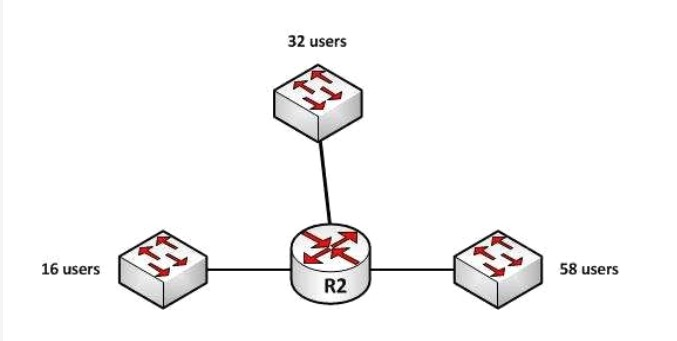# CISCO CCNA 200-301 Q138

You are working with an Internet Service Provider (ISP) as network manager. A corporate client approaches you to lease a public IP subnet that can accommodate 250 users. You have assigned him the 192.25.27.0 subnet.

What subnet mask should be assigned to this IP address so that it can accommodate the number of users required by the corporate client?

A. 255.255.255.0
B. 255.255.255.128
C. 255.255.255.224
D. 255.255.255.252

Explanation:
The 192.25.27.0 subnet should be assigned the subnet mask of 255.255.255.0 to accommodate 250 users. This subnet mask can accommodate a maximum of 254 hosts. The number of hosts that can reside on a subnet can be calculated using the formula 2^n – 2 = x, where n is equal to the number of hosts bits in the mask and x is the resulting number of hosts. 2 is subtracted from the results to represent the two address, the network ID and the broadcast address, that cannot be assigned to computers in the subnet. Since the 255.255.255.0 mask leaves 8 bits at the end of the mask, the formula will be 2^8 – 2, which is 256 – 2, which equals 254.

In situations where the same subnet mask must be used for multiple interfaces on a router, the subnet mask that is chosen must provide capacity sufficient for the largest number of hosts on any single interface while also providing the required number of subnets. For example, in the diagram below, the three interfaces on the router R2 have 16, 32 and 58 users respectively on each interface:If each interface must have the same subnet mask, the subnet mask would need to be one that yields at least 58 addresses to support the interface with the highest host count and yields at least 3 subnets as well.

If the chosen classful networks were 128.107.4.0/24, the correct mask would be 255.255.255.192. Since the mask is currently 255.255.255.0 (/24), by borrowing 2 bits to /26 or 255.255.255.192, we will get 4 subnets (2^2 = 4) and each subnet will yield 62 hosts (2^6 – 2 = 62).

With a subnet mask of 255.255.255.128, the 192.25.27.0 subnet can accommodate only 126 hosts. The mask 255.255.255.128 leaves 7 host bits in the mask and when we plug that into the formula we get 2^7 – 2, which equals 126.

With a subnet mask of 255.255.255.224, the 192.25.27.0 subnet can accommodate only 30 hosts. The mask 255.255.255.224 leaves 5 host bits in the mask and when we plug that into the formula we get 2^5 – 2, which equals 30.

With a subnet mask of 255.255.255.252, the IP address 192.25.27.24 can accommodate only two hosts. The mask 255.255.255.252 leaves 2 host bits in the mask and when we plug that into the formula we get 2^2 – 2, which equals 2.